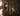🌀
What is a pure function?
July 28, 2019· 3 min readOne of the first things you will encounter when learning functional programming is the idea of pure functions. Functional programming is all about combining small pieces of code together to form a large vision, and pure functions help us do just that.

I'm going to use javascript in this article because its the most accessible and popular language in the world. Meaning you can most likely take these ideas and start using them immediately.

A Pure Function

A function is pure if it passes two simple tests:

1. Given the same input, it will always return the same output.
2. It has no side effects.

Let’s take a look these two rules.

Given the same input, it will always return the same output

Here's a pure function:

const cowSay = phrase => `The cow says \${phrase}`

We say cowSay is pure because if you call it with the string 'moo' , it will always return the string 'The cow says moo', no matter how many times you call it.

for (let i = 1; i =< 100; i++) {
const says = cowSay("moo")
console.log(`\${i}: \${says}`)
}
// Output
// 1: The cow says moo
// 2: The cow says moo
// 3: The cow says moo
// 4: The cow says moo
// 5: The cow says moo
// 6: The cow says moo
// ... and so on up to 100

A more complex example that calculates the price of items in an order:

const order = {
items: [
{ name: "bandage", price: 1.25 },
{ name: "coffee", price: 3.4 },
{ name: "board game", price: 12.99 }
]
}

// calculateTotal is pure because if you give it the same items array// 1 million times, it always will return the same totalconst calculateTotal = items => items.reduce((item, acc) => acc + item.price, 0)

const total = calculateTotal(order.items)

console.log(`Your total is \${total}`) // Your total is 17.64

It has no side effects

A function has a side effect if it modifies state outside of itself.

These three functions below (double, addOne, log) all perform side-effects:

let state = 2

// Effect-ful
const double = () => {
state = state * 2
}

const addOne = () => {
state = state + 1
}

const log = () => console.log(state)

// Program
log() // 2
double()
log() // 5

double()
double()
log() // 20

Take note that the log function above performs a side-effect as well, it logs to the console. The console is a stateful output device who’s state is what has been displayed to the user.

This immediately begs the question, ”Well then, where do we put side effects?!? This program works perfectly fine, why would I change it?" There are a couple answers to these questions, but the easiest is to just clearly separate them.

Let’s re-write the example above by separating side-effect and pure functions:

let state = 2

// Effect-ful
function change(newState) {
state = newState
}

function log() {
console.log(state)
}

// Pure
const add = number => number + 1

const double = number => number * 2

// Program
log() // 2
change(double(state))
log() // 5

change(double(state))
change(double(state))
log() // 20

Now, the logic of the program (pure functions) is separated from the things that change state (side-effect functions). We do this because side-effects are often un-predictable. They rely on external systems that we don’t control. If we isolate our side effects, we can create programs that are more predictable.

Here's a great answer on Stack Overflow if you want to go deeper into managing side-effects.

Conclusion

I hope that was helpful! Pure functions have helped me write cleaner code and understand my code better when it comes time to debug my applications. Learning functional programming can be overwhelming. Hopefull this was helpful to you in understanding this small part of functional programming.